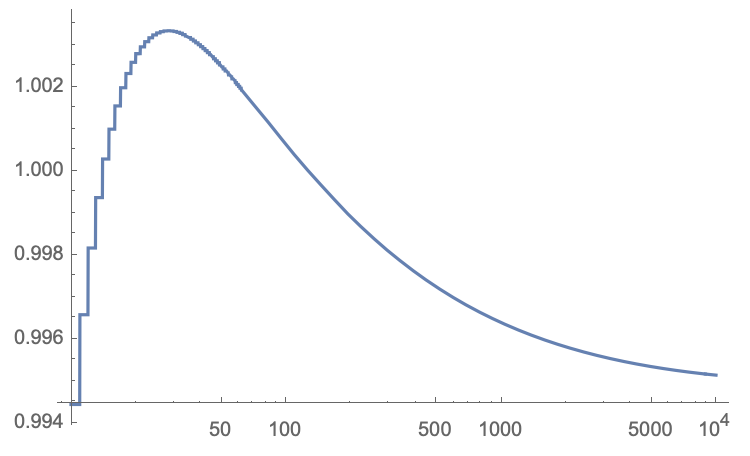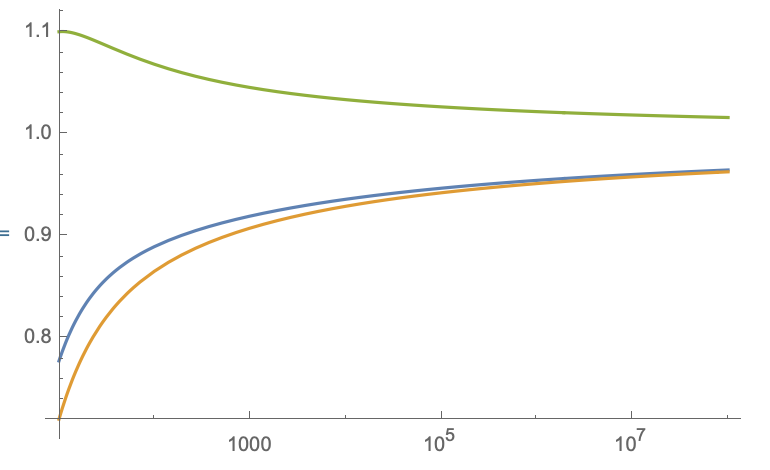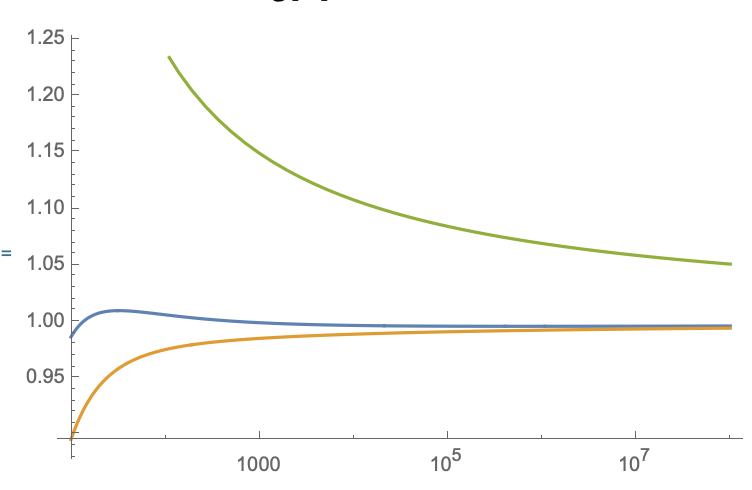# Value of $c$ such that $\lim_{n\rightarrow\infty}\sum_{k=1}^{n-1}\frac{1}{(n-k)c+\log(n!)-\log(k!)}=1$

What is the value of $$c$$ such that $$\lim_{n\rightarrow\infty}\sum_{k=1}^{n-1}\frac{1}{(n-k)c+\log(n!)-\log(k!)}=1?$$ Numerically, it seems that the answer is $$c=\log 2$$. But I'd like to see a reason why.

• Hi, welcome to MO. That looks interesting, but if you could give us more details about HOW you arrived to this expression, maybe it would help people here to answer your question. Clearly, it didn't grow out of thin air. – Amir Sagiv Dec 4 '18 at 3:45

## 2 Answers

UPDATE

I tried to evaluate the sum numerically for large $$n$$ and what I find does not support the conclusion I give below, that the large-$$n$$ limit equals 1 independent of $$c$$. Here is a plot for $$c=\log 2$$, obtained with Mathematica. I am uncertain about the numerical stability, but if this plot is to be trusted the $$n\rightarrow\infty$$ limit for $$c=\log 2$$ is below unity. Apologies for my mistaken conclusion, I might delete this answer, but perhaps it can still serve a purpose.One way to proceed is as follows. For large $$n$$ I approximate the sum $$S(c,n)=\sum_{k=1}^{n-1}\frac{1}{(n-k)c+\log(n!)-\log(k!)}$$ by an integral over $$k$$ and I approximate the factorials $$n!$$ and $$k!$$ by Stirling's formula, so instead of the sum $$S(c,n)$$ I evaluate the integral

$$I(c,n)=\int_{1}^{n-1} \frac{1}{(n+1/2)\ln n-(k+1/2)\ln k+(c-1) (n-k)}\,dk.$$

Now for the large-$$n$$ asymptotics of $$I(c,n)$$, I first note the upper bound $$I(c,n)\leq\int_{1}^{n-1} \frac{1}{(n+1/2)\ln n-(k+1/2)\ln n+(c-1) (n-k)}\,dk$$ $$\qquad\qquad=\frac{\ln (n-1)}{c-1+\ln n}\rightarrow 1\;\;\text{for}\;\;n\rightarrow\infty.$$

For a lower bound I expand the integrand around $$k=n$$, $$I(c,n)\geq \int_{1}^{n-1} \frac{1}{(n-k) \left(c+\frac{1}{2n}+\ln n\right)}\,dk$$ $$\qquad\qquad=\frac{2 n \ln (n-1)}{2 c n+2 n \ln n+1}\rightarrow 1\;\;\text{for}\;\;n\rightarrow\infty.$$

The plot shows $$I(c,n)$$ (blue) and the upper and lower bounds, for $$c=\ln 2$$ (at the left) and for $$c=0.1$$ (at the right). Notice that the lower bound is actually quite accurate already for moderately large $$n$$.• For what it is worth, I would recommend against deleting. – Yaakov Baruch Dec 5 '18 at 10:24
• For the lower bound write $S(n,c)=\sum_{k=1}^{n-1}\frac{1}{k\,c+\log(n!)-\log((n-k)!)}$ and use $\log(n!)-\log((n-k)!)\leq k\log(n)$ to find that $$S(n,c)\geq \frac{H_{n-1}}{c+\log(n)}\;\;,$$ where $H_{n-1}$ is the $(n-1)-$th harmonic number. Thus $\liminf S(n,c)\geq 1$ (independent of $c\geq 0$), as originally asserted. – esg Dec 13 '18 at 19:00

I think, that $$c=\gamma=0.57721..$$ (Euler-Mascheroni), because $$\sum_{k=1}^{n-1} \frac{1}{(n-k) c + \ln \Gamma(n+1)-\ln \Gamma(k+1)} = \\ \sum_{m=1}^{n-1} \frac{1}{\ln(\frac{ e^{m c}\ \Gamma(n+1)}{\Gamma(n-m+1)})} \sim \sum_{m=1}^{n-1} \frac{1}{m \ \ln (e^{c}\ n)} = \\ \frac{1}{\ln (e^{c}\ n)} H_{n-1} \sim \frac{1}{\ln (e^{c}\ n)} (\ln n +\gamma)$$ where in the second line I changed the summation variable to $$m=n-k$$ and used $$\frac{\Gamma(x+a)}{\Gamma(x+b)}\sim x^{a-b}$$ for large $$x$$.$$H_n$$ is the $$n$$-th Harmonic number and its asymptotic expansion for large $$n$$ is $$H_{n}\sim \ln n +\gamma +O(n^{-1}).$$ All expansions/definitions can be found at Wikipedia or at https://dlmf.nist.gov/

• You seem to use $\frac{\Gamma(n-1)}{\Gamma(n-m+1)}\sim n^m$ for $1\le m \le n-1$. But $n-m$ (or even $n$) is not arbitrarily large relative to $m$ in most of that range. Notice that you reach the same (likely erroneous) conclusion that the limit is $1$ regardless of $c$, as in the previous answer. – Yaakov Baruch Dec 5 '18 at 23:10
• correct me if I'm wrong, but my impression is that this calculation expands the numerator to first order around $k=n$, to evaluate the sum $$\sum_{k=1}^{n-1}\left[\left(n-k) (c+\psi ^{(0)}(n+1)\right)\right]^{-1}=\frac{\gamma+\psi ^{(0)}(n)}{c+\psi ^{(0)}(n+1)},$$ with $\psi$ the polygamma function, which tends to 1 in the limit $n\rightarrow\infty$ irrespective of $c$. – Carlo Beenakker Dec 6 '18 at 15:08